Author: Oscar Cronquist Article last updated on June 07, 2022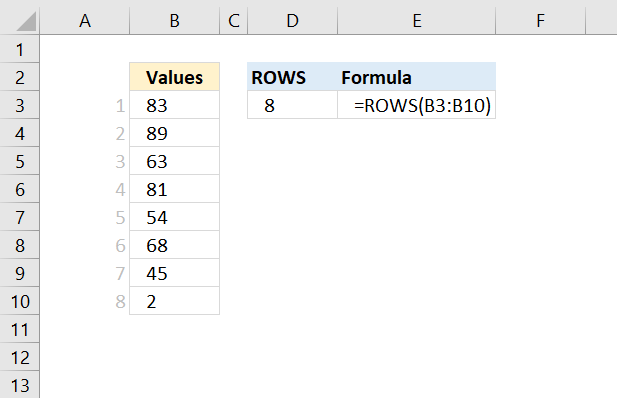The ROWS function allows you to calculate the number of rows in a cell range.

The example above shows that cell range B3:B10 contains 8 rows.

ROWS(array)

## 2. ROWS Function Argument

 array Required. A cell range for which you want to calculate the number of rows.

## 3. ROWS Function Example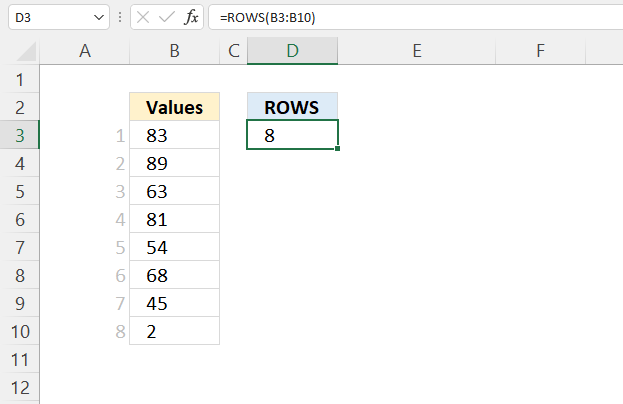Formula in cell D3:

=ROWS(B3:B10)

## 4. Count rows in an array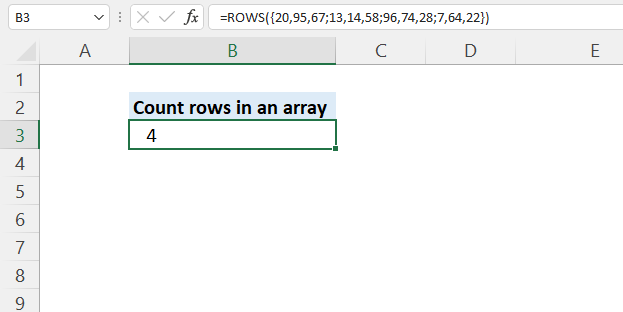The ROWS function also calculates the number of rows in an array.

Formula in cell B3:

=ROWS({20,95,67; 13,14,58; 96,74,28; 7,64,22})

The array has four rows. The ; (semicolon) character is a row delimiting character in an array.

## 5. Count rows based on a condition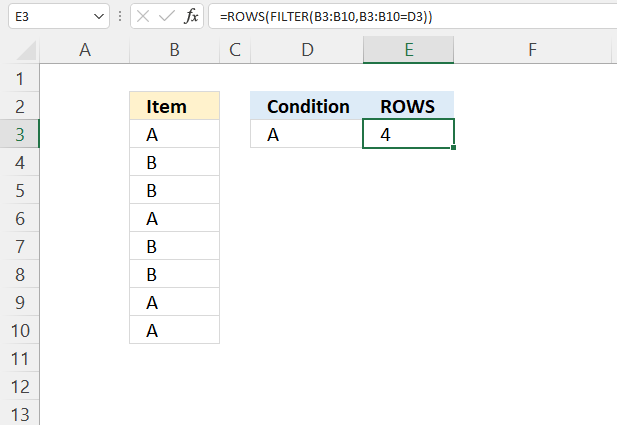Formula in cell E3

=ROWS(FILTER(B3:B10,B3:B10=D3))

### Explaining formula

#### Step 1 - Logical expression

The equal sign lets you compare value to value, it is also possible to compare a value to an array of values. The result is either TRUE or FALSE.

B3:B10=D3

becomes

{"A"; "B"; "B"; "A"; "B"; "B"; "A"; "A"}="A"

and returns

{TRUE; FALSE; FALSE; TRUE; FALSE; FALSE; TRUE; TRUE}.

#### Step 2 - Filter values based on a condition

The FILTER function gets values/rows based on a condition or criteria.
FILTER(arrayinclude, [if_empty])

FILTER(B3:B10,B3:B10=D3)

becomes

FILTER({"A"; "B"; "B"; "A"; "B"; "B"; "A"; "A"}, {TRUE; FALSE; FALSE; TRUE; FALSE; FALSE; TRUE; TRUE})

and returns

{"A"; "A"; "A"; "A"}.

#### Step 3 - Count rows

ROWS(FILTER(B3:B10,B3:B10=D3))

becomes

ROWS({"A"; "A"; "A"; "A"})

and returns 4.

## 6. Count rows based on a list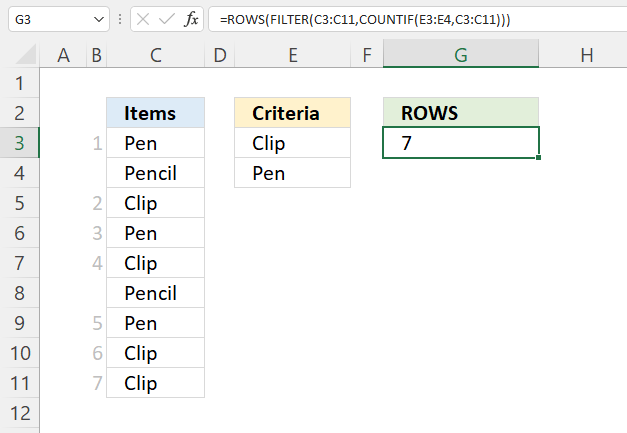Formula in cell F3:

=ROWS(FILTER(C3:C11,COUNTIF(E3:E4,C3:C11)))

### Explaining formula

#### Step 1 - Which values equal any item in the list

The COUNTIF function counts the number of cells that meet a given condition.
COUNTIF(rangecriteria)

COUNTIF(E3:E4, C3:C11)

becomes

COUNTIF({"Clip"; "Pen"},{"Pen"; "Pencil"; "Clip"; "Pen"; "Clip"; "Pencil"; "Pen"; "Clip"; "Clip"})

and returns {1; 0; 1; 1; 1; 0; 1; 1; 1}.

#### Step 2 - Filter values based on array

The FILTER function gets values/rows based on a condition or criteria.
FILTER(arrayinclude, [if_empty])

FILTER(C3:C11,COUNTIF(E3:E4,C3:C11))

becomes

FILTER({"Pen"; "Pencil"; "Clip"; "Pen"; "Clip"; "Pencil"; "Pen"; "Clip"; "Clip"}, {1; 0; 1; 1; 1; 0; 1; 1; 1})

and returns

{"Pen"; "Clip"; "Pen"; "Clip"; "Pen"; "Clip"; "Clip"}.

#### Step 3 - Count rows

ROWS(FILTER(C3:C11,COUNTIF(E3:E4,C3:C11)))

becomes

ROWS({"Pen"; "Clip"; "Pen"; "Clip"; "Pen"; "Clip"; "Clip"})

and returns 7.

## 7. Count rows in a delimited string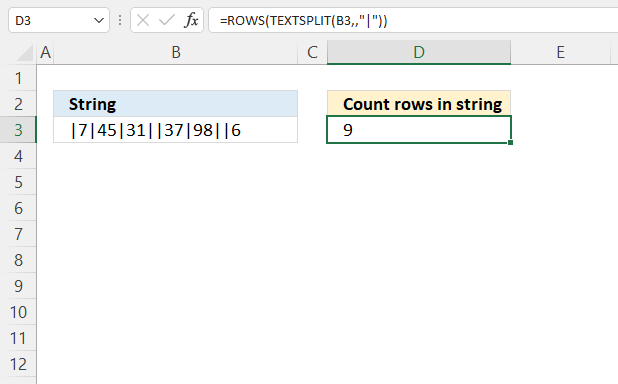The formula in cell D3 counts delimited values in a string located in cell B3, you can use any character or string a s a delimiting character.

Excel 365 dynamic array formula in cell C3:

=ROWS(TEXTSPLIT(B3,,"|"))

### Explaining formula

#### Step 1 - Split string using a given delimiting character

The TEXTSPLIT function lets you split a string into an array across columns and rows based on delimiting characters.

TEXTSPLIT(B3,,";")

becomes

TEXTSPLIT("|7|45|31||37|98||6",,";")

and returns

{""; "7"; "45"; "31"; ""; "37"; "98"; ""; "6"}.

The semicolon is a delimiting character in arrays, however, they are determined by your regional settings. In other words, you may be using other delimtiing characters.

#### Step 2 - Count rows

ROWS(TEXTSPLIT(B3,,";"))

becomes

ROWS({""; "7"; "45"; "31"; ""; "37"; "98"; ""; "6"})

and returns 9. The values in the array are arranged vertically. An horizontal array would be using commas, like this: {"", "7", "45", "31", "", "37", "98", "", "6"}.

## 8. Count rows in multiple cell ranges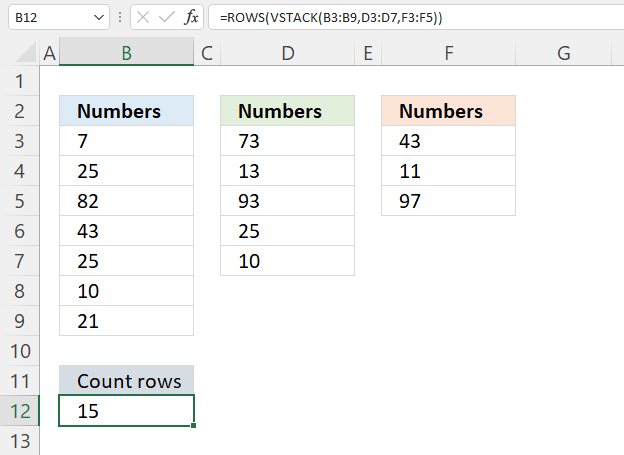This example demonstrate how to count rows in three different sized cell ranges simultaneously and return total rows.

Formula in cell B12:

=ROWS(VSTACK(B3:B9,D3:D7,F3:F5))

### Explaining formula

#### Step 1 - Join arrays

The VSTACK function combines cell ranges or arrays, it joins data to the first blank cell at the bottom of a cell range or array.
VSTACK(array1,[array2],...)

VSTACK(B3:B9, D3:D7, F3:F5)

becomes

VSTACK({7; 25; 82; 43; 25; 10; 21},{73; 13; 93; 25; 10; 65; 91},{43; 11; 97; 61; 4; 45; 91})

and returns

{7; 25; 82; 43; 25; 10; 21; 73; 13; 93; 25; 10; 65; 91; 43; 11; 97; 61; 4; 45; 91}.

#### Step 2 - Calculate rows

ROWS(VSTACK(B3:B9,D3:D7,F3:F5))

becomes

ROWS({7; 25; 82; 43; 25; 10; 21; 73; 13; 93; 25; 10; 65; 91; 43; 11; 97; 61; 4; 45; 91})

and returns 15.